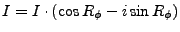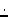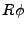Next: Input Cards Up: usermanual Previous: Output Description   Contents

# SUBTRREFPHA

In this chapter the processing of step SUBTRREFPHA is described.

This step requires the steps INTERFERO and COMPREFPHA for obvious reasons. In this step the reference phase of a mathematical body (ellipsoid) is subtracted from the complex interferogram. This is done by complex multiplication with the conjugated, written symbolically as follows:(Y1)

Where I is the complex interferogram,denotes pointwize multiplication, andis the reference phase for a certain point.

Subsections

Leijen 2009-04-14